﻿ 相控阵线圈的相位计算Member of Mulan DX Club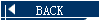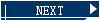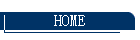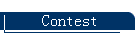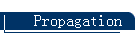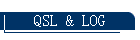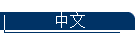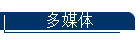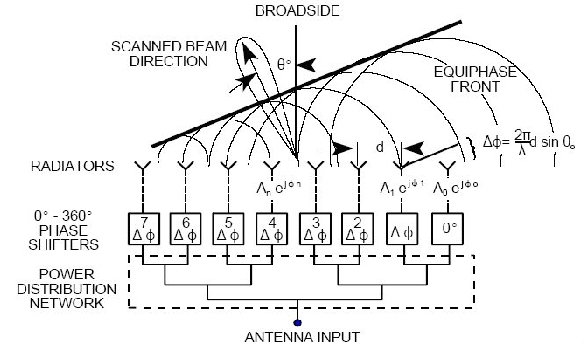Δφ=2π/Λ*d*SIN(Θ)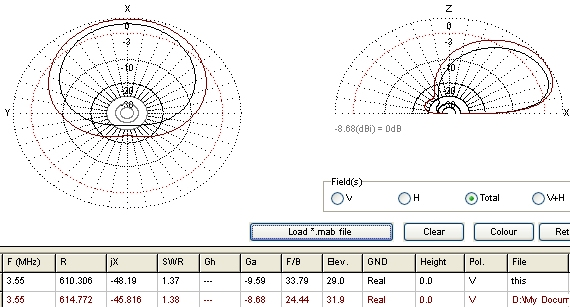### Endfire Array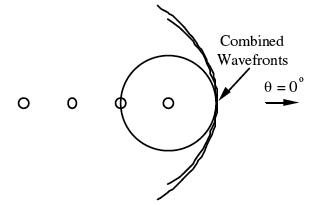$varphi = frac{{2pi }}{lambda }scos left( theta right) + alpha$$0 = frac{{2pi }}{lambda }scos left( 0 right) + alpha$$alpha = - frac{{2pi }}{lambda }s$$AF = left| {frac{{sin left( {frac{{nvarphi }}{2}} right)}}{{sqrt n sin left( {frac{varphi }{2}} right)}}} right|quad {rm{where}}quad varphi = frac{{2pi }}{lambda }sleft( {cos left( theta right) - 1} right)$${rm{generally}}quad s = frac{lambda }{4}quad quad quad {rm{therefore}}quad quad quad varphi = frac{pi }{2}left( {cosleft( theta right) - 1} right)$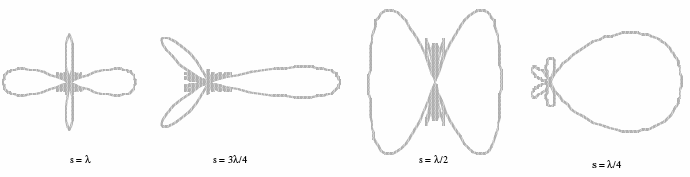φ=2π/Λ*S*COS(Θ)+α

 BA4ALC/BA5CW 2014-09-30